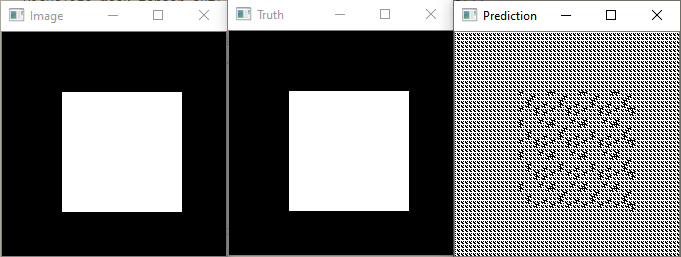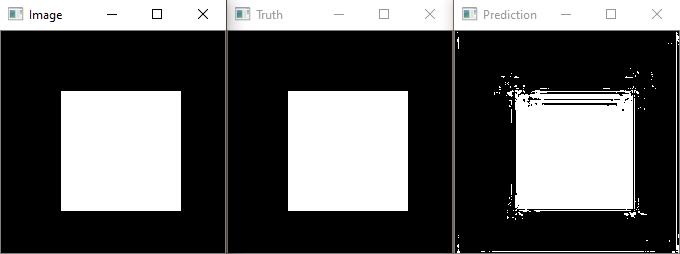# FCN8s Implementation - Network not learning

Hi everybody!

I am new to PyTorch and attempting to implement and train a VGG16-based FCN8s architecture for binary semantic segmentation from scratch, based on the FCN paper by Long et al. I have successfully done so in Keras+TF before and I can’t figure out why the loss is not decreasing in this case (constantly around ~0.693 with minor variations).

My FCN Module is as follows:

``````class FCN(nn.Module):

def __init__(self, num_classes=1):
super().__init__()

self.num_classes = num_classes

self.block1 = self.conv2(3, 64)
self.block2 = self.conv2(64, 128)
self.block3 = self.conv3(128, 256)
self.block4 = self.conv3(256, 512)
self.block5 = self.conv3(512, 512)

# Convolution Block combining 2 Convolutions followed by ReLu Activations and Max-Pooling
def conv2(self, num_in, num_out):
return nn.Sequential(
nn.Conv2d(num_in, num_out, kernel_size=3, stride=1, padding = 1),
nn.ReLU(inplace=True),
nn.Conv2d(num_out, num_out, kernel_size=3, stride=1, padding = 1),
nn.ReLU(inplace=True),
nn.MaxPool2d(2, 2)
)

# Convolution Block combining 3 Convolutions followed by ReLu Activations and Max-Pooling
def conv3(self, num_in, num_out):
return nn.Sequential(
nn.Conv2d(num_in, num_out, kernel_size=3, stride=1, padding = 1),
nn.ReLU(inplace=True),
nn.Conv2d(num_out, num_out, kernel_size=3, stride=1, padding = 1),
nn.ReLU(inplace=True),
nn.Conv2d(num_out, num_out, kernel_size=3, stride=1, padding = 1),
nn.ReLU(inplace=True),
nn.MaxPool2d(2, 2)
)

def forward(self, input): # Input: 224 x 224 x 3

# VGG16 ENCODER

x = self.block1(input) # -> (112 x 112 x 64)
x = self.block2(x) # -> (56 x 56 x 128)
x = self.block3(x) # -> (28 x 28 x 256)
pool3 = x
x = self.block4(x) # -> (14 x 14 x 512)
pool4 = x
x = self.block5(x) # -> (7 x 7 x 512)

x = nn.Conv2d(512, 4096, kernel_size=3, stride=1, padding= 1)(x) # -> (7 x 7 x 4096)
x = nn.ReLU()(x)

x = nn.Conv2d(4096, 4096, kernel_size=1, stride=1)(x) # -> (7 x 7 x 4096)
x = nn.ReLU()(x)

x = nn.Conv2d(4096, self.num_classes, kernel_size=1, stride=1)(x) # -> (7 x 7 x Classes)

# DECODER

# 2x Upsampling
x = nn.ConvTranspose2d(self.num_classes, self.num_classes, kernel_size=2, stride = 2,bias=False)(x)
pool4 = nn.Conv2d(512, self.num_classes, kernel_size=1)(pool4)
x += pool4

# 2x Upsampling
x = nn.ConvTranspose2d(self.num_classes, self.num_classes, kernel_size=2, stride = 2,bias=False)(x)
pool3 = nn.Conv2d(256, self.num_classes, kernel_size=1)(pool3)
x += pool3

# 8x Upsampling
x = nn.ConvTranspose2d(self.num_classes, self.num_classes, kernel_size=2, stride = 2,bias=False)(x)
x = nn.ConvTranspose2d(self.num_classes, self.num_classes, kernel_size=2, stride = 2,bias=False)(x)
x = nn.ConvTranspose2d(self.num_classes, self.num_classes, kernel_size=2, stride = 2,bias=False)(x)

x = F.sigmoid(x)
return x
``````

My goal was to feed only one image to the network for multiple epochs, trying to overfit this image. To this end, I generated a binary image of size [3,224,224] showing a white square on black background. The target is essentially the same image, but of size [1,224,224]. Most of the time, the prediction after some epochs (between 10 and 100) is either black/white only or a repeating pattern. In any case, the values of the prediction are very close to 0.5, indicating that the network is basically guessing. The best result the network could achieve thus far looked as follows:I have tried the following steps, but with no success:

• Use one-hot encoding by adapting the target and changing the num_classes to 2, resulting in a prediction of shape [2,224,224]. I also changed the Sigmoid function to Softmax.

• Different optimizers (SGD, Adam) with different learning rates, momentum

• Different Loss-functions (BCELoss, BCEWithLogitsLoss, CrossEntropyLoss)

• Replacing ConvTranspose2D with Upsampling+Conv2D+ReLu

I suspect that either my model is faulty or I am missing an important PyTorch-related detail that I am not aware of. Simply using this UNet model instead of my FCN by only changing the line `net=FCN()` to `net=UNet()` yields a good result and the BCELoss decreases from 0.7 to 0.56 within 10 epochs (although the values in the prediction are between 0.48 and 0.97 instead of close to 0 and 1, which seems a bit fishy).

So my training procedure should in general be fine. Anyway, here’s the code I use:

``````net = FCN()
net.train()
epochs = 100
loss_func = nn.BCELoss()
optimizer = optim.SGD(net.parameters(), lr=0.01)

inp = np.zeros((224, 224, 3))
inp[60:180, 60:180, 0:3] = 1
inp = inp*2-1
totens = transforms.ToTensor()
inp = totens(inp)
inp = torch.unsqueeze(inp, dim=0).float()

truth = np.zeros((224, 224, 1))
truth[60:180, 60:180, 0] = 1
truth = totens(truth)
truth = torch.unsqueeze(truth, dim=0).float()

for i in range(0,epochs):
out = net(inp)
loss = loss_func(out, truth)
loss.backward()
optimizer.step()
print("Epoch " + str(i) + " Loss: " + str(loss.item()))
``````

I appreciate any comments that guide me in the right direction. I have looked at the code for quite a while and seem to be blind to the mistake.

Here’s the prediction of the UNet I get after some epochs:One issue with the code is that you are re-initializing some layers on your `forward` method.
You would usually initialize all modules in the `__init__` method and assign them to internal attributes, as you have done with `self.block1` etc.
However, all `nn.Conv2d` and `nn.ConvTranspose2d` calls in your `forward` will use new and randomly initialized modules, which won’t be trained.

I’m not sure, if that’s your use case, but I would guess not.

Initialize these modules in your `__init__` and rerun your code.

Also, instead of `sigmoid` + `nn.BCELoss`, I would recommend to use raw logits + `nn.BCEWithLogitsLoss` for more numerical stability.

You’re absolutely right! I found that out just before I saw your reply, the network seems to learn properly now. Looks like I missed the line

• `nn.Parameter` - A kind of Tensor, that is automatically registered as a parameter when assigned as an attribute to a `Module` .

when reading the Blitz Tutorial. Endet up costing me about two days of work to figure that out…

I have switched to a prediction of size 2 (because I want to do binary segmentation) along Dim1 + `nn.CrossEntropyLoss`, but I will try your suggestion and see if it can further improve the results! I guess sticking with BCELoss would save some resources in the decoder.

The only thing that is not quite clear to me is why the model seems to only have ~790k trainable parameters instead of the ~2M it had in Keras, despite using the same number of filters in each layer (obtained using `sum(p.numel() for p in net.parameters() if p.requires_grad)` )

Do you still see the different number of parameters after fixing the re-initialization issue?
If so, could you post the Keras implementation so that we could compare these models?

Yes, but that was just a mistake on my side.
I was using a 3x3 kernel further down the PyTorch network instead of a large 7x7 kernel in Keras. By replacing it, I get about the number of parameters I was expecting.

Thanks for your help!